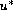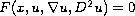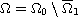Electron. J. Diff. Eqns., Vol. 2006(2006), No. 124, pp. 1-12.

### Convexity of level sets for solutions to nonlinear elliptic problems in convex rings Paola Cuoghi, Paolo Salani

Abstract:
We find suitable assumptions for the quasi-concave envelopeof a solution (or a subsolution)of an elliptic equation(possibly fully nonlinear) to be a viscosity subsolution of the same equation. We apply this result to study the convexity of level sets of solutions to elliptic Dirichlet problems in a convex ring.

Submitted June 23, 2005. Published October 11, 2006.
Math Subject Classifications: 35J25, 35J65.
Key Words: Elliptic equations; convexity of level sets; quasi-concave envelope.

Show me the PDF file (243K), TEX file, and other files for this article.Paola Cuoghi Dipt. di Matematica Pura e Applicata Universitá degli Studi di Modena e Reggio Emilia via Campi 213/B, 41100 Modena -Italy email: pcuoghi@unimo.it Paolo Salani Dipt. di Matematica "U. Dini" viale Morgagni 67/A, 50134 Firenze, Italy email: salani@math.unifi.it